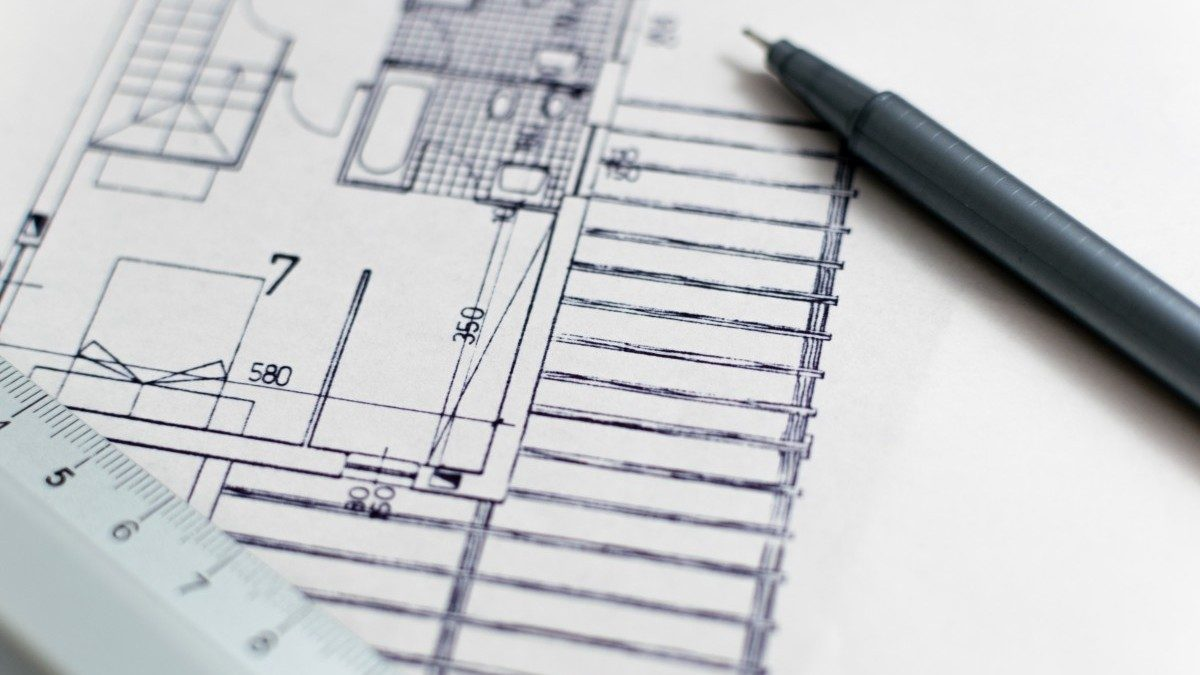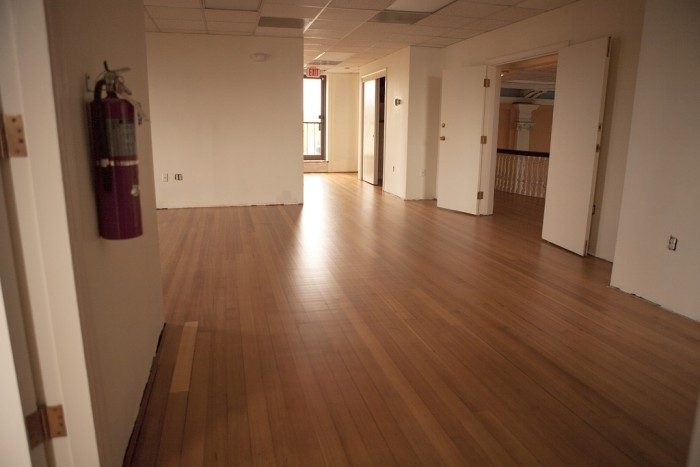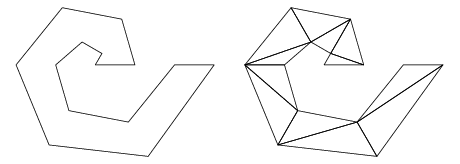# Square Footage Calculator

Created by Álvaro Díez and Steven Wooding
Reviewed by Bogna Szyk and Jack Bowater
Last updated: Feb 03, 2022

Square footage calculator is an easy tool that enables you to calculate an area in square feet as well as perform conversions, such as finding out how many square feet are in an acre. In this article, we are going to explain how to calculate square footage and understand the square footage formula.

Moreover, we will instruct you on how to find the square footage from the area given in some other units; that includes converting sq into sq ft (square inches to square feet), sq m to sq ft (square meters to square feet) and acres to sq ft (acres to square feet). In fact, we're feeling so generous we will even talk about how to measure the square footage of a house.

## What is a square foot?

A square foot or sq ft is an imperial unit of area that is widely used in the United States, Canada, China, and the United Kingdom. Its symbol is a simple square with a vertical line bisecting it (), commonly used in many branches, such as architecture, real estate, and interior space plans. It may also be occasionally written as a square with a slash through it. Its abbreviation is commonly written as sqft or sq ft.As a unit of area, it has a magnitude equivalent to the area of a square with sides of 1 foot. This size makes it helpful to talk about the area of everyday objects such as a house (typically 500-1000 sq ft), a room (~100 sq ft) and even an A4 piece of paper (0.65 sq ft) without having to use either very big or very small numbers. There exist, obviously, other units of area that can express the same magnitude as the sq ft and might even be more suitable for very small objects (like the square inch), very big objects (like the acre), or to simply to communicate with the rest of the world by using the standardized SI/Metric units (whose default unit of area is the square meter).

You will see soon how to convert from square meters to square feet, from square inches to square feet, etc... But for now, let's talk about some situations in which you might want to calculate the square footage of something using a simple square footage formula. These situations include selling, leasing, renting, or buying a house or a room; building a shed or a garage for your car; or maybe even when painting a room. In all these situations, our square footage calculator will help you. Although, for the last three, we recommend looking at our paint calculator.

We often also want to calculate the volume in cubic yards, especially while planning to purchase landscape material. To help you with such a situation, you can use our square feet to cubic yards calculator.

## Calculate square footage (sq ft) from other units.

Let's now see how to calculate square footage when you already know the area in different units. This is the most simple use of the square footage calculator. To use this conversion feature, you could use the area conversion tool on this website or simply follow the following steps:

1. In the square footage calculator find the Area tab and select units you want to convert from,
2. You can now enter the value of the area in those units,
3. Click again on the unit switcher and select square feet (sq ft),
4. Automatically, the calculator will show the value in sq ft.

It is also possible to convert in the other direction by applying this method, i.e., from a different unit, like square meters to square feet. We will now show some examples of conversions to and from square footage.

## How to convert square meters to square feet?

For example, we might want to know how big a 100 m2 (sqm) expressed in sq ft. In other words, we would want to convert from sq m to sq ft and find out the square footage of that house, which is:

• 100 sqm * 10.764 sq ft/sqm = 1076.391 sq ft

## How to convert acres to sq ft?

The procedure of converting square inches to square feet or from acres to sq ft is the same as converting from square meters to square feet. In the following examples, you will find the most common of these conversions: how many square feet are in an acre.

• 1 acre * 43 560 sq ft/acre = 43 560 sq ft
• 30 sq in * 0.00694 sq ft/sqin = 0.208333 sq ft

Intuitively, this process can also be done in reverse. Therefore, we can not only find square footage when we know its value expressed in the other units but also find an area in any units from a given square footage.

## How to use the square footage calculator

Before we get into the nitty-gritty of how the calculator works and what is the square footage formula, it's useful to know how to use the calculator, and what each of the components mean. With the "One room/area" option selected, the square footage calculator is composed of the following fields:

1. Shape - Select the room/area shape from rectangle, square, circle, triangle, hexagon or octagon
2. Measurements - Various measurements of the room, which change depending on the shape selected
3. Quantity - Enter the number of rooms/areas that have the same shape and measurements
4. Area - Combined square footage of all the spaces as input above
5. Unit price - Price (in the local currency) per square foot
6. Total cost - Combined monetary value of the spaces described above

To use the calculator is as simple as setting the known values and letting the system calculate the rest. This means that you can use this calculator to compute the price per square foot of a property if you know the total price and total square footage.

If you select the option "Multiple rooms/areas" at the top of the calculate, you can enter the measurements for up to ten rooms or areas and get a grand total at the bottom of the calculator of the square footage. For complex room layouts, divide up the room into simple shapes, such as rectangles, squares, etc., and enter each one as a separate room/area. If you've entered a unit price, you'll also get the total cost.

## How to calculate areas and what is the square footage formula?

Technically, it is not correct to talk about the square footage formula; we should rather talk about area formula. However, as long as we understand that a square footage formula is just another way to say that we will take an area formula and apply it using square feet, I think we can all agree the precise technical term doesn't matter much here.

The square footage formula is different depending on the shape of the object we want to calculate the area for. The simplest and most common case is the square, or, more generally, the rectangle. For these polygons, the formula is as simple as taking the length of two adjacent sides in feet and multiplying them together. Since we are working with a rectangle, we know that taking two next sides is the same as taking the height and the width of it. If we name these two sides A and B, the other two necessarily must have the same dimensions as A and B, respectively.• square_footage = length_A * length_B

This might sound like a simple mathematical formula, but it is precisely how to measure the square footage of a rectangular room in real life. We just need to measure two consecutive sides in feet and multiply the values together. This square footage formula is, in fact, the same as the one used in the area of a rectangle calculator, but, in case of this calculator, used to find square footage instead of calculating polygons.

Not all rooms are perfect squares or rectangles, and it becomes more tricky to calculate square footage in such a situation. If the room is almost rectangular, one can get a pretty good approximation just by considering it as a rectangular and performing a similar calculation as we have seen before. But when the shape of the room (or whatever you want to measure) is far from rectangular, we definitely recommend using the corresponding Omni calculator for that shape,e.g., it could be a triangle area calculator, the area of a circle calculator, or any other shape.

Note that these calculators for geometrical shapes can calculate areas in almost any unit you would wish for. This means that you can convert from acres to sq ft, to square meters, to square feet, and so on, using the same procedure that we have described in the previous section.

## How to calculate square footage pricing

Once again, we tackle a widespread issue that, despite the complications which might arise in real life, has some rather simple maths beneath it. The calculations of square footage pricing are, mathematically, the simple division of the price of a specific property by its total square footage. In everyday life, this value changes significantly depending on such factors as a location of the property, intended or potential use, and so on. It is nonetheless a useful quantity to evaluate the value of a particular house or property.Before we talk a bit more about the usefulness of this measurement, let's take a look at how we can use this calculator when square footage pricing comes into play. First, make sure that the "One room/area" option is selected at the top of the calculator. The area can be calculated in the previous steps or can also be inputted by the user. Then either the price per sq ft or the total cost should be provided to obtain the other value. Let's look at a complete example:
1. Input the size of the property's (or property chunk's) width and length in your desired units,
2. Input the number of properties/chunks of the property with the size input above,
3. [only if you didn't follow the first two steps] Input the total area in your desired units,
4. Input the total pricing of the property,
5. Obtain the square footage pricing as dollars (or your local currency) per sq ft.

This is an example of one of the most straightforward scenarios, but it is very representative of the typical uses of this square footage calculator. We think that it is essential not only to know how to calculate square footage or how to measure square footage but also to know what you can do with those values once you get them.

When it comes to square footage pricing, its usefulness relies on the fact that it allows comparing properties (mostly houses) of different sizes and prices. It's the equivalent of performance per dollar charts of computer parts, for example. In this manner, one could compare a 1500 sq ft with a 500 sq ft and know which one represents a better real state option, looking beyond just the price or the size of them. In fact, this can be used with any other area unit with or without converting from square meters to square feet or acres to square feet - every time we want to make a fair comparison.

## How to measure the square footage of a house or property

We have touched previously on how to calculate and measure square footage in a simple situation like a rectangular room/house or a circular and triangular... But there are situations in which the shape of the property we want to measure square footage from, is a bit more complicated than that. In those cases there are two options: (1) using the area of a polygon calculator and other similar calculators by Omni (we can recommend the hexagon / octagon area calculators or (2) get creative, as we're gonna do now.For very complicated and irregular shapes, there is no simple recipe for how to measure the square footage of a house using just one tool. However, we can use a simple trick to achieve the appropriate result. We can try to decompose the area into several small pieces that have a regular shape, for which we can calculate square footage. To each of those pieces, we apply the square footage formula corresponding to its polygonal shape (different Omni Calculators will prove useful here). If we then add up the result from every piece, we will obtain the total square footage of the house, no matter its shape.

There is a chance that the house will have rounded corners of curvy shapes (they are very popular nowadays), and this might seem impossible to do. But in reality, one can get a very good approximation using a straight line that goes to the middle of the curved ones or, if we want to be more precise, many small polygons. It could be time-consuming, but it simultaneously can be as accurate as one needs.

## Final remarks about square footage

If you live in North America or one of the other few countries that still use the Imperial system, talking about square footage might be natural. Nevertheless, it is important to remember that most of the countries in the world use the metric system, which measures area in square meters. Having a basic, approximate idea of what the conversion from square meters to square feet is, can be valuable in the communications across different countries. A good "ballpark" value that's easy to remember is that 10 sq ft ~ sqm - to convert from sq m to sq ft, we just need to add a zero at the end of the number.

Another important thing to remember is that a board foot is not a unit of area, although it sounds very similar. In fact, it is a unit of volume. Therefore, there is no conversion possible between them, and they are not equivalent by any means. So even though how many acres are in square feet? is a valid question to ask, asking how many board feet are in a square foot? is like saying how many tables are in a swimming pool. But, if you wish to know more about board feet or you feel like calculating some volumes for fun (we don't judge, people have different hobbies), then feel free to check the board foot calculator.

Álvaro Díez and Steven Wooding
One or multiple rooms
One room/area
Shape
RectangleWidth
ft
Length
ft
Quantity
Area
ft²
Cost of materials
Unit price
$/ ft² Total cost$
People also viewed…

Addiction calculator tells you how much shorter your life would be if you were addicted to alcohol, cigarettes, cocaine, methamphetamine, methadone, or heroin.

### Pizza size

This calculator will help you make the most delicious choice when ordering pizza.

### Rebar calculator

Use the rebar calculator to find out how many rebars are needed for a concrete slab and how much it will cost you.

### Stairs

The stair calculator helps you design comfortable stairs: it finds the stair stringer length, as well as the rise and run of individual steps.